Thank you for visiting nature.com. You are using a browser version with limited support for CSS. To obtain the best experience, we recommend you use a more up to date browser (or turn off compatibility mode in Internet Explorer). In the meantime, to ensure continued support, we are displaying the site without styles and JavaScript.

Universal statistics of the knockout tournament

Abstract

We study statistics of the knockout tournament, where only the winner of a fixture progresses to the next. We assign a real number called competitiveness to each contestant and find that the resulting distribution of prize money follows a power law with an exponent close to unity if the competitiveness is a stable quantity and a decisive factor to win a match. Otherwise, the distribution is found narrow. The existing observation of power law distributions in various kinds of real sports tournaments therefore suggests that the rules of those games are constructed in such a way that it is possible to understand the games in terms of the contestants' inherent characteristics of competitiveness.

Introduction

Competition is a ubiquitous form of social interaction for distributing limited resources among a number of individuals, often regarded as the opposite of cooperation. Competition has been a main tenet in economics where a perfectly competitive equilibrium is proven Pareto-efficient as long as there are no externalities and public goods. Moreover, the notion of natural selection in biological evolution is often understood as proving competition ‘natural’. For these reasons, although competition results in growing tension across a society, most people have taken it for granted as an organising principle of our society.

Recently, Deng et al.1 claimed universal power-law distributions of scores and prize money by observing various kinds of sports such as tennis, golf, football, badminton and so on. According to their extensive data analysis, the probability to find scores or prize money greater than k always decays as a power law P>(k) ~ k−(γ−1) with an exponential cutoff where the power-law exponent γ − 1 ranges between 0.01 and 0.39 depending on sports. In addition, they presented a knockout-tournament model to explain the observations. This is an intriguing approach since the most organised forms of competition are usually found in sports. It is also popular to run a knockout tournament, consisting of successive rounds where only a winner in each fixture progresses to the next round, because it is an efficient procedure to find who is the best with a small number of fixtures. In other words, Deng et al. hinted a direct connection between the structure of competition and its consequences. Physicists have already recognised sports as a fruitful research field: Statistics of athletic records has been pioneered by Gembris et al.2 and Wergen et al.3, for example and there have been attempts to even predict the limiting performances in the long run4. Sports ranking combinatorics has also been considered by Park and Newman5,6. If we are to understand the dynamics governing high achievements in sports careers, in particular, one famous theory along this direction is called the Matthew “rich get richer” effect7,8,9: It says that a higher position leads to a better chance to progress further in career, resulting in an extremely skewed distribution. The spatial Poisson process to model this effect indeed explains such behaviour with γ ≤ 1, which is found in some empirical data sets. However, we should point out that many factors of competition are hidden in the probability of progress and that the stochastic process is totally indifferent to individual characteristics as written in Ecclesiastes: “the race is not to the swift, but time and chance happenth to them all”.

In this work, we instead focus on statistical analysis of a specific system of competition, i.e., the knockout tournament among inhomogeneous participants. Our main point is that a large part of statistics is universal in the sense that it is independent of most details of the game but already determined by the tournament structure. Let us consider a player's number of wins denoted by n, for example. When the tournament has been finished, the distribution of n denoted by P(n) is always an exponentially decreasing function of n. It is a purely geometric property of the tournament tree independent of any details of the game, loosely mapped to the critical percolation on a binary tree10. If the prize money is highly skewed towards the best players, similarly to real sports tournaments, one can assume that the prize money kn after winning n rounds is also an exponential function of n, that is, kn ~ zn (Fig. 1). Combining these two, one finds that the distribution P(k) ~ k−γ with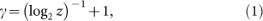and this mechanism belongs to combination of exponentials according to Newman11. If z gets very large, γ converges to unity, yielding P(k) ~ k−1. As z → 1, on the other hand, γ diverges because P(k) approaches the distribution function of n, which is an exponential function. In fact, if z < 2, the total amount of prize money gets unbounded as the number of contestants grows, which means that the organiser of this tournament has a risk of bankruptcy. This explains why kn has to be such a rapidly increasing function of n and we see that the feasible range of γ is between one and two. Moreover, if there is a typical number of prize winners, z is effectively very large, driving γ to unity. This is a simple prediction for a single tournament. In other words, this analysis corresponds to gathering data of prize money distributed over many tournaments without identifying who was who. The actual statistics collected in this way, however, will not be very interesting to us and it is usually more meaningful to consider individual-based statistics: Even for a team sport, each team may be regarded as an individual. It is notable that Deng et al. resolve this problem by introducing the notion of ranks, belonging to individuals and also by assuming that a player's winning probability against another is a function of their rank difference. Following this approach, we will see how our simple prediction in equation (1) can be reproduced on average in the individual-based statistics.

Results

Decisiveness of competitiveness

Imagine a tournament with N = 2m contestants to construct a simple binary tree. Each person is assigned a real number r, which we refer as competitiveness instead of a rank and reserve the latter term for denoting an outcome of competition, which may or may not reflect an individual's genuine competitiveness depending on how much luck comes into play. By defining r as a real number, the competitiveness is automatically assumed to be transitive, which means that if contestant A is more competitive than B who is more competitive than C, then A is also more competitive than C. Since we can always rescale the highest competitiveness as unity and the lowest one as null without loss of generality, the real number r belongs to a closed interval from zero to one.

Under total uncertainty about the contestants, we may assume as our initial condition that the distribution of r is uniformly random at the starting point. We thus denote the initial probability density distribution of r as p0(r) = 1 with normalisation. Then, we introduce a function f(r, r′) that defines the probability for a contestant with competitiveness r to defeat another with r′. As was done by Deng et al.1, it can be assumed to be a function of xrr′ only and it is plausible in such a case that f(x) is a nondecreasing function of x [−1, 1] with f(x) + f(−x) = 1. In words, the former condition means that a more competitive player has a higher probability to defeat a less competitive player, whereas the latter condition is merely a simple reflection of the trivial fact that one of the two players must win, irrespective of their values of r. Let us check some examples of f(x).

Perfect resolution

One of the simplest choices iswhere Θ is the Heaviside step function. This means that the competitiveness decides the outcome deterministically. In Methods, we have derived the following nonlinear recursive relation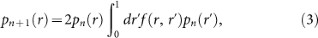where pn(r) means the distribution of r after the nth round. With the Heaviside step function, this equation is solvable at any arbitrary n and we obtain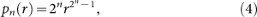with a corresponding cumulative distribution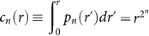. As explained in Methods, cn(r) is identical to the winning chance for the contestant with r at the (n + 1)th round, denoted by wn(r), when we have chosen the step function in equation (2).

We can extract various useful information from this probability density function. For example, the average competitiveness after the nth round is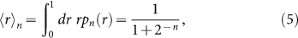and therefore the width of pn(r) decreases as σ ~ 2n. A contestant with r passes the nth round but not the next one with probability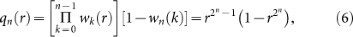where we have used wk = ck and the sum over n is normalised to unity for any r between zero and one. The average prize money for this person with r can thus be calculated as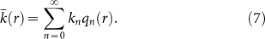As shown in Fig. 2, qn has a peak atand the summations above can be approximated as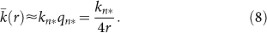If kn = zn, it means that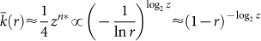in the vicinity of r = 1. Note that we have approximated r as unity at the denominator of equation (8). Therefore, Zipf's plot shows a power law with slope −log2 z, leading to P(k) ~ k−γ with γ = (log2 z)−1 + 1 due to the relationship between Zipf's plot and P(k)12. This exactly coincides with equation (1) derived for a single tournament. We have numerically performed tournaments and the results confirm validity of our analysis as shown in Fig. 3, where the numerical calculations of c5(r) and 〈rn agree perfectly with the analytic results. The detailed procedure of our simulation is explained in Methods.

Imperfect resolution

As an opposite extreme case, let us consider a situation where individual competitiveness is totally irrelevant to the outcome of a match and only luck decides. In other words, we assume a constant function f(x) = 1/2. If we start from p0 = 1, the winning chance here is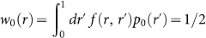. Note that w0 is not identical to the cumulative distribution any more. The next round has a distribution p1(r) = 2w0(r)p0(r) = 1 and this pattern is repeated all the way leading to pn(r) = 1 for every n. It is also straightforward to obtain the same result by substituting the constant f(x) = 1/2 into the recursive equation (3). The resulting P(k) is just the most likely distribution of the prize money among the N players, so the maximum entropy principle tells us to maximizewhere the first term is Shannon entropy and μ represents a Lagrangian multiplier for constraining the average prize money. When H is maximised, it does not change under variation in P(k) to the first order and we thus have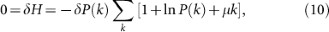which leads us to P(k) ~ exp(−k/kc) with a characteristic scale kc.

This implies a tendency that P(k) usually exhibits a power law with an exponent close to unity but that randomness makes the tail shorter. Suppose that f(x) has a finite resolving power, quantified by a characteristic width Γ over which f(x) rapidly increases. The Heaviside step function corresponds to a limiting case of Γ → 0. We can predict the followings when Γ is finite but sufficiently small: At the beginning of the competition, the width σ of pn(r) is much greater than Γ, so f(x) effectively serves as a step function. The above analysis shows that σ decreases as 2n so it becomes comparable with Γ after ν ~ log2(1/Γ) rounds. Thereafter, the decrease of σ slows down. Finally, whenafter many rounds, the survivors' competitiveness is irrelevant and the outcomes are mostly determined by pure luck. Therefore, a natural guess for P(k) would be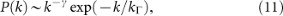with kΓ ~ O(zν) and γ in equation (1). This functional form is confirmed in our numerical simulations (Fig. 4). This distribution can also be derived from the maximum entropy principle as in equation (10) but with an additional constraint on Σk ln k13,14, which corresponds to the total number of fixtures in this context. The above argument can be pursued further by employing the following f(x):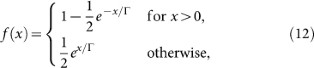where the exponential functions make it possible to explicitly evaluate the integral. Then, the winning chance is given as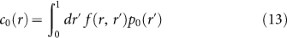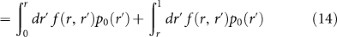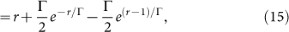which approaches c0(r) = r as Γ → 0 and c0(r) = 1/2 as Γ → ∞, as expected. As above, this yields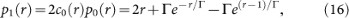which is normalised to unity as. This result is quite suggestive, because equation (16) modifies equation (4) at n = 1 by adding O(Γ) whenand subtracting the same amount when[Fig. 5(a)]. In short, p0(r) becomes flatter when r is close to 0 or 1. If we take one step further, the low-r correction becomes less important and we find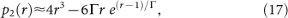where we have left only the dominant correction of O(Γ) [Fig. 5(b)]. For general n, the result up to the correction of O(Γ) is inductively found as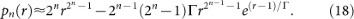This implies that the finite resolution is most noticeable among highly competitive players with, whereas the story looks similar to the case of perfect resolution when (1 − r) is small but still much larger than Γ.

Stability of competitiveness

We have assumed that competitiveness is each individual's inherent characteristic, which changes in a much longer time scale compared to outcomes of competition and we relate the latter to ranks. The idea is that although a contestant's rank fluctuates over tournaments, it will correctly reflect her true competitiveness in the long run. Even if the competitiveness may interact with actual tournament results, it will usually be related to a cumulative measure of performance that mainly reflects low-frequency, i.e., long-term behaviour. For example, we have calculated the Kendall tau rank correlation coefficient15, denoted by τ, to see how the accumulated amounts of prize money change their relative positions between two successive tournaments (Fig. 6). If a certain pair of contestants keep their relative positions, they are said to be concordant and discordant otherwise. The coefficient τ is defined as the number of concordant pairs minus that of discordant pairs, divided by the total number of possible pairs. Beginning with the same initial amount of money for every contestant, which is set to zero, we run fifty tournaments in a row, accumulating the prize money for each individual. A contestant's accumulated money from a series of tournaments determines her performance in the next tournament in such a way that r = (Ni)/(N − 1) is assigned to the contestant when she has the ith largest accumulated amount. The relative positions of two equal amounts are random. In spite of this variability, the ranks of the accumulated money get stabilised after 20 or 30 tournaments in all the cases considered (Fig. 6) and the resulting P(k) is almost identical to the static-r case for each Γ. Still, one may ask what happens if their time scales approach each other so that a current rank directly affects performance at the next tournament, provided that the tournaments are regular events. Even if an individual's rank fluctuates over time, it might still be possible for this correlation between successive tournaments to reproduce the power-law tail part of P(k). In fact, this question is not really well-posed because a knockout tournament leaves many contestants' ranks undetermined except a few prize winners and this is the fundamental advantage of a knockout tournament. We nevertheless suppose that a player's competitiveness at the next time step is a nondecreasing function of the current performance, say, rt+1 = R(nt), where nt is the number of wins in the tournament at time t and R is a nondecreasing function between zero and one. Since r determines how many rounds the contestant can go through, the distribution of nt+1 is essentially a function of nt. The situation is actually boring because the same contestant wins the first place all the time, but we may exclude this exceptional contestant from our consideration. We begin with noting that any tournament results in a distribution of nt as, which is the initial distribution of the next tournament at time t + 1. The corresponding cumulative portion of contestants with results below nt is thus. As above, if f(x) is the Heaviside step function with f(0) = 1/2, the chance to win the first round for a contestant that passed nt rounds at the previous tournament is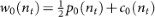. The first term represents the probability to meet an opponent with the same nt and the factor of one half originates from f(0). The distribution of nt at the next round is p1(nt) = 2w0(nt)p0(nt). We can repeat this procedure to obtain a general expression as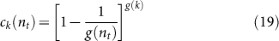with g(x) ≡ 2x. By definition, we have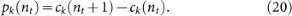If k is not very small, pk(nt) converges to a certain function of yknt with a maximum around y ≈ 0 [Fig. 7(a)]. The conditional probability to reach k and stop there for given nt is found aswith[Fig. 7(b)]. We observe that qk(nt) can also be described as a certain function V(y) when. Moreover, we find thatfor any nt. In other words, the time series {nt ≥ 0} can be roughly described as a biased random walk towards the origin. Since this holds true for anyone, each contestant's average result will be rapidly equalised by the bias so we predict that the probability distribution P(k) will be narrow. This prediction is well substantiated by numerical results shown in Fig. 8, where P>(k) is drawn in a semi-log plot. Therefore, in terms of the time scale of competitiveness, the power-law shape of P(k) is observable when competitiveness changes much more slowly compared to the frequency of tournaments.

Discussion

In summary, we have investigated statistics resulting from knockout tournaments. It is basically the rules of the game that define competitiveness, so the distribution of prize money is dependent on how much the rules take individual competitiveness as a decisive and stable factor. But other details of the game are found irrelevant and the statistics is universal in this sense. More specifically, if competitiveness is a static parameter and any tiny difference of it can be distinguished by the rules, the distribution is predicted to take a power-law shape P(k) ~ k−γ with γ close to unity. If the difference is indistinguishable below a certain resolution limit Γ, we find an exponential cutoff at the tail, whose location is a function of Γ. We have also argued that the distribution P(k) becomes narrow again when competitiveness changes with a time scale comparable to the frequency of tournaments. In this respect, the broad distributions observed across many sports suggest that their rules are already stabilised in such a way that one can readily compare contestants' competitiveness in a consistent way over a long time span and that the result of competition sensitively reflects the difference indeed. Since our analysis relates certain internal parameters of a given tournament such as z and Γ to the final distribution of prize money, which is somewhat more easily accessible, it will an interesting question to verify such detailed relationships directly on empirical grounds.

Methods

Recursive relation for pn(r)

In case of perfect resolution, i.e., f(r, r′) = Θ(rr′), it is straightforward to obtain the winning chance for the contestant with r at the first round of the tournament as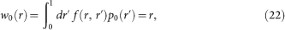where p0(r′) = 1. This happens to be identical to the cumulative distribution c0(r) and it represents the simple fact that the contestant with r should meet an opponent with r′ < r in order to win and progress to the next round. When the first round has been finished, the distribution of their competitiveness is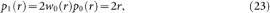which is again normalised to unity. The factor of two in front is needed because the number of survivors has become one half of N. Note that we have used independence between a player's competitiveness and her opponent's in equation (23), which is the case when the initial condition contains no correlations in competitiveness. As in the first round, the corresponding cumulative distribution,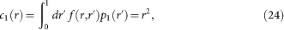is identical to the winning chance w1(r) at the second round. In the same way, the distribution after the second round is p2(r) = 2w1(r)p1(r) = 4w1(r)w0(r)p0(r) = 4r3 and so on. For general f(r, r′), we can use essentially the same argument to derive the following nonlinear recursive relation: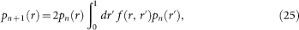which is explicitly solvable for a few special cases as above.

Numerical procedures

First, we generate a tournament tree with N = 2m contestants at the terminal nodes and assign to each of them a real random number r inside the unit interval as competitiveness. One may require the minimum and maximum of the random numbers to be strictly zero and one, respectively, but it does not make a visible difference when N is large enough. The resulting uncorrelated random number sequence {r1, r2, …, rN} means absence of a seeding process, so number one and number two seeds may face each other in the first round. Second, when two contestants A and B meet with rA and rB, respectively, we draw a random number ρ [0, 1) and choose A as the winner of this fixture if ρ < f(rA, rB) and choose B otherwise. This is repeated for every match in this first round and the winner progresses to the parent node. When we have filled all the parent nodes with 2m−1 winners, the second round starts among them in the same way as before. As the tournament proceeds round by round, the number of survivors decreases rapidly until the final winner is left alone after the mth round. Each player defeated at the nth round receives prize money zn−1, whereas the final winner acquires zm. When a tournament is over, we start a new one with randomly shuffling {r1, r2, …, rN} at the terminal nodes, so that the competitiveness is identified as an individual characteristic preserved across the tournaments. We have performed 104 shuffles, hence the same number of tournaments, to obtain statistical averages for each ri with N = 212.

References

1. Deng, W., Li, W., Cai, X., Bulou, A. & Wang, Q. A. Universal scaling in sports ranking. New J. Phys. 14, 093038 (2012).

2. Gembris, D., Taylor, J. G. & Suter, D. Sports statistics: Trends and random fluctuations in athletics. Nature (London) 417, 506 (2002).

3. Wergen, G., Volovik, D., Redner, S. & Krug, J. Rounding effects in record statistics. Phys. Rev. Lett. 109, 164102 (2012).

4. Radicci, F. Universality, limits and predictability of gold-medal performances at the Olympic Games. PLoS ONE 7, e40335 (2012).

5. Park, J. & Newman, M. E. J. A network-based ranking system for US college football. J. Stat. Mech. P10014 (2005).

6. Park, J. Diagrammatic perturbation methods in networks and sports ranking combinatorics. J. Stat. Mech. P04006 (2010).

7. Petersen, A. M., Jung, W.-S., Yang, J.-S. & Stanley, H. E. Quantitative and empirical demonstration of the Matthew effect in a study of career longevity. Proc. Natl. Acad. Sci. USA 108, 18–23 (2011).

8. Petersen, A. M., Penner, O. & Stanley, H. E. Methods for detrending success metrics to account for inflationary and deflationary factors. Eur. Phys. J. B 79, 67–78 (2011).

9. Petersen, A. M., Jung, W.-S. & Stanley, H. E. On the distribution of career longevity and the evolution of home-run prowess in professional baseball. EPL 83, 50010 (2008).

10. Baek, S. K., Minnhagen, P. & Kim, B. J. Percolation on hyperbolic lattices. Phys. Rev. E 79, 011124 (2009).

11. Newman, M. E. J. Power laws, Pareto distributions and Zipf's law. Contemp. Phys. 46, 323–351 (2005).

12. Kim, B. J. & Park, S. M. Distribution of Korean family names. Physica A 347, 683–694 (2005).

13. Baek, S. K., Bernhardsson, S. & Minnhagen, P. Zipf's law unzipped. New J. Phys. 13, 043004 (2011).

14. Visser, M. Zipf's law, power laws and maximum entropy. New J. Phys. 15, 043021 (2013).

15. Kendall, M. A new measure of rank correlation. Biometrika 30, 81–89 (1938).

Acknowledgements

We are indebted to Petter Minnhagen for introducing us to this problem. We thank Korea Institute for Advanced Study for providing computing resources (KIAS Center for Advanced Computation, Abacus System) for this work. B.J.K. was supported by the National Research Foundation of Korea (NRF) grant funded by the Korea government (MEST) (No. 2011-0015731).

Author information

Authors

Contributions

B.S.K. & B.J.K. designed research, performed research, wrote, reviewed and approved the manuscript. I.G.Y. & H.J.P. performed the numerical and statistical analysis of the data.

Ethics declarations

Competing interests

The authors declare no competing financial interests.

Rights and permissions

Reprints and Permissions

Baek, S., Yi, I., Park, H. et al. Universal statistics of the knockout tournament. Sci Rep 3, 3198 (2013). https://doi.org/10.1038/srep03198

• Accepted:

• Published:

• DOI: https://doi.org/10.1038/srep03198